# 限定修饰符const

2016/10/22 22:02

const int a = 1;
int const a = 1;

const关键字修饰变量a，表示a是一个整型变量，且这个变量的值是不可改变的。这两个定义的作用一样，编译后变量a分配在只读数据段中，这个数据段在一块只读的内存区域中，任何对这块区域的操作都是非法的。

#include <stdio.h>

int main(void)
{
int const a = 100;
a = 200;
return 0;
}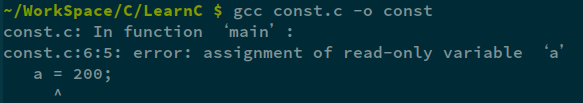## const关键字修饰指针--在指针定义之前

const关键字在指针变量的定义之前，如下所示：

int const a = 100;
const int *p;  //指针指向的内容不能改变
//等同于 int const *p;
p = &a;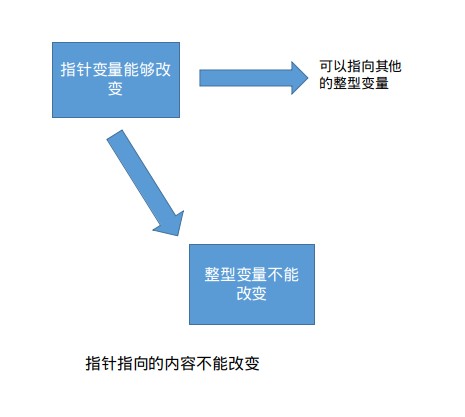#include <stdio.h>

int main(void)
{
int a = 100;
int b = 200;
const int *p;
p = &a;
printf("a = %d\n", *p);
*p = 200;   //错误，指针所指向的内容(通过*p方式)不能改变
p = &b;     //正确，指针本身可以改变
printf("a = %d\n", *p);
return 0;
}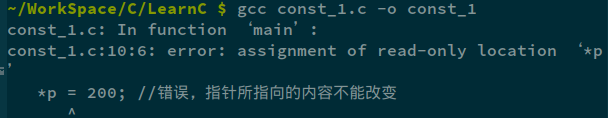## const关键字修饰指针--在指针定义之中

const关键字在指针变量的定义之中：

int a = 100;
int * const p = &a;  //指针本身不能改变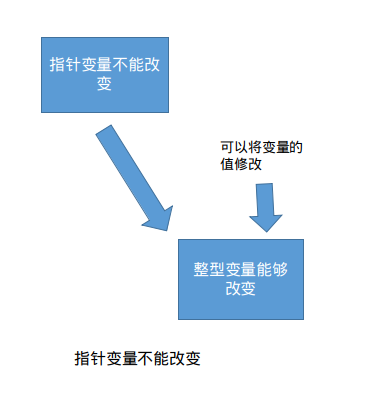#include <stdio.h>

int main(void)
{
int a = 100;
int b = 200;
int  * const p = &a;
printf("a = %d\n", *p);
*p = 200;   //正确，指针所指向的内容(通过*p方式)可以改变
//p = &b;     //指针本身不可以改变
printf("a = %d\n", *p);
return 0;
}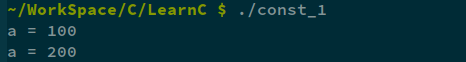## const关键字修饰指针--在指针定义之前和定义之中

const int a = 100;
int const * const p = &a; //指针和指针指向的内容都不能改变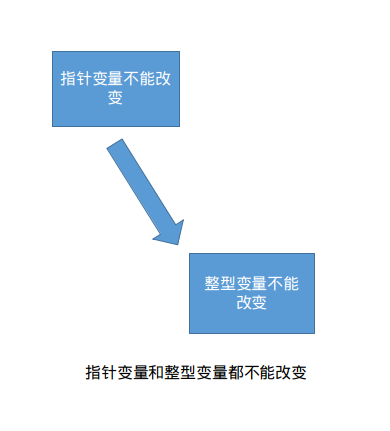#include <stdio.h>

int main(void)
{
int a = 100;
int b = 200;
int const * const p = &a;
printf("a = %d\n", *p);
*p = 200;   //错误，指针所指向的内容不能改变
p = &b;     //错误，指针本身不可以改变
printf("a = %d\n", *p);
return 0;
}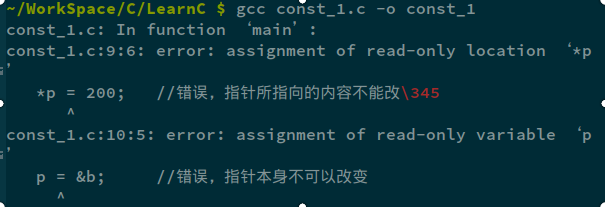int a = 100;
const int *p;
p = &a;  //常量指针指向一个普通变量

const int a = 100;
int *p = &a;  //普通指针不能指向一个常量变量

## 使用const关键字的意义

• 合理的只用cosnt关键字，可以使编译器保护那些不希望被改变的参数，防止被意外修改，可以较少bug的出现。
• 关键字const是为了告诉用户这个参数的引用目的。例如一个函数的参数是const char*，这样用户就可以放心的传给它char*或者const char*指针，而不必担心指针所指的内存区域被改写
• 通过给编译器一些附加信息，是const关键字也许能产生更紧凑的代码

#include <stdio.h>

//将所有空格替换为"_",失败返回NULL
char * replace(char *str)
{
char *p;
if(str == NULL)
return NULL;
p = str;
while(*p != '\0'){
if(*p == ' ')
*p = '_';  //进行字符串的替换
p++;
}
return p;
}

int main(void)
{
char *p = "hello world and china\n";
if(replace(p) != NULL)
printf("the string : %s\n", p);
return 0;
}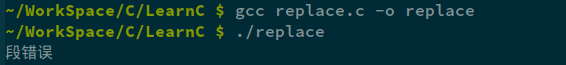#include <stdio.h>

//将所有空格替换为"_",失败返回NULL
const char * replace(const char *str)  //使用const修饰变量
{
const char *p;
if(str == NULL)
return NULL;
p = str;
while(*p != '\0'){
if(*p == ' ')
*p = '_';  //进行字符串的替换
p++;
}
return p;
}

int main(void)
{
const char *p = "hello world and china\n"; //将源字符串声明为const变量
if(replace(p) != NULL)
printf("the string : %s\n", p);
return 0;
}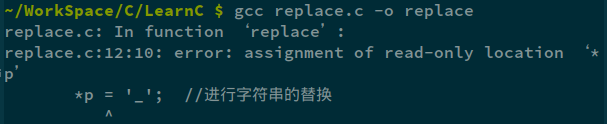0
0 收藏

### 作者的其它热门文章0 评论
0 收藏
0